# Clustering[Evaluation]

### 0. 背景

• 内部评估的方法：通过一个单一的量化得分来评估算法好坏；该类型的方法
• 外部评估的方法：通过将聚类结果与已经有“ground truth”分类进行对比。要么通过人类进行手动评估，要么通过一些指标在特定的应用场景中进行聚类用法的评估。不过该方法是有问题的，如果真的有了label，那么还需要聚类干嘛，而且实际应用中，往往都没label；另一方面，这些label只反映了数据集的一个可能的划分方法，它并不能告诉你存在一个不同的更好的聚类算法。

### 1. 内部评估的方法

• 那些高分的算法不一定可以适用于高效的信息检索应用场景；
• 这些评估方法对某些算法有倾向性，如k-means聚类都是基于点之间的距离进行优化的，而那些基于距离的内部评估方法就会过度的赞誉这些生成的聚类结果。

Davies-Bouldin 指标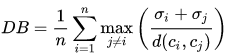Dunn 指标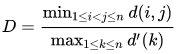• 类间距离$d(i,j)$可以是任意的距离测度，例如两个类别的中心点的距离；
• 相似的，类内距离$d'(k)$可以以不同的方法去测量，例如类别$k$中任意两点之间距离的最大值。

Silhouette 系数

### 2. 外部评估方法

Purity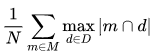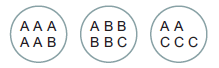Rand 测度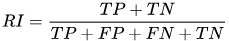TP表示相同label在同一个聚类类别中计算得$TP=C_5^2+C_4^2+[C_3^2+C_2^2]=20$;
TP+FP表示样本被预测为正的结果$TP+FP=C_6^2+C_6^2+C_5^2=40$;$FP=40-20=20$;
FN表示相同label在不同聚类类别中$FN=[C_5^1C_1^1]_{A_{12}}+[C_5^1C_2^1]_{A_{13}}+[C_1^1C_2^1]_{A_{23}}+[C_1^1C_4^1]_{B_{12}}+[C_1^1C_3^1]_{C_{23}}=24$
TN表示不同label在不同聚类类别中$TN=[C_5^1C_4^1]_{A_1B_2}+[C_5^1C_1^1]_{A_1C_2}+[C_5^1C_3^1]_{A_1C_3}+[C_1^1C_1^1]_{B_1A_2}+[C_1^1C_1^1]_{B_1C_2}+[C_1^1C_2^1]_{B_1A_3}+[C_1^1C_3^1]_{B_1C_3}+[C_1^1C_3^1]_{A_2C_3}+[C_4^1C_2^1]+{B_2A_3}+[C_4^1C_3^1]_{B_2C_3}+[C_1^1C_2^1]_{C_2A_3}=72$

F-测度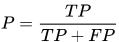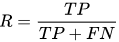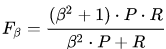$\beta=0$时，就是准确率，这时候召回率的影响为0，通过增加$\beta$可以调节两个值的影响程度

Jaccard 指数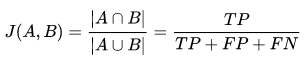Dice 指数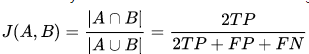Fowlkes-Mallows 指数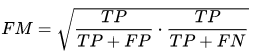mutual information

$p_{UV}(i,j)=\frac{|u_i\cap v_j|}{N}$表示一个样本点属于$U$$u_i$类别和$V$$v_j$类别的概率。同样的，$p_U(i)=\frac{u_i}{N}$表示一个样本点属于$U$$u_i$类别的概率，$p_V(j)=\frac{v_j}{N}$类似。其中$N$表示数据集中样本点个数。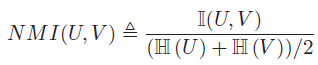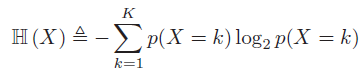K表示类别个数，$p(X = k)$表示一个样本属于类别k的概率

### 3. 聚类趋势

Hopkins statistic

• $X$表示在$d$维空间上的$n$个数据点，先对其随机采样$m\ll n$个数据点$x_i$。同时随机生成$m$个均匀分布的数据点集合$Y$。现在定义两个距离测度：
• $u_i$表示$y_i\in Y$$X$中最近的点的距离；
• $w_i$表示$x_i\in X$$X$中最近的点的距离。
然后计算：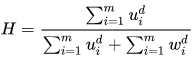通过这个定义，均匀采样的数据通常倾向得到值为0.5，而可以聚类的数据可以得到接近1的值。然而当数据值包含一个高斯分布的时候，同样也能得到值1，因为该统计是测量偏离均匀分布的程度，而不是呈现多模态的特性，从而使得该统计量在实际应用中基本没用（因为真实数据从来都不是均匀分布的）。

 - Machine learning, a probabilistic perspective
 - .Evaluation_and_assessment

posted @ 2018-03-16 17:27  仙守  阅读(822)  评论(0编辑  收藏  举报